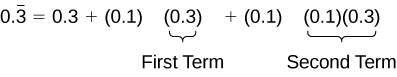# 13.4 Series and their notations  (Page 5/18)

 Page 5 / 18
$\begin{array}{l}{\left(\frac{1}{2}\right)}^{10}=\frac{1}{1\text{,}024}\hfill \\ {\left(\frac{1}{2}\right)}^{20}=\frac{1}{1\text{,}048\text{,}576}\hfill \\ {\left(\frac{1}{2}\right)}^{30}=\frac{1}{1\text{,}073\text{,}741\text{,}824}\hfill \end{array}$

As $n$ gets very large, ${r}^{n}$ gets very small. We say that, as $n$ increases without bound, ${r}^{n}$ approaches 0. As ${r}^{n}$ approaches 0, $1-{r}^{n}$ approaches 1. When this happens, the numerator approaches $\text{\hspace{0.17em}}{a}_{1}.$ This give us a formula for the sum of an infinite geometric series.

## Formula for the sum of an infinite geometric series

The formula for the sum of an infinite geometric series with $-1 is

$S=\frac{{a}_{1}}{1-r}$

Given an infinite geometric series, find its sum.

1. Identify ${a}_{1}$ and $r.$
2. Confirm that $–1
3. Substitute values for ${a}_{1}$ and $r$ into the formula, $S=\frac{{a}_{1}}{1-r}.$
4. Simplify to find $\text{\hspace{0.17em}}S.$

## Finding the sum of an infinite geometric series

Find the sum, if it exists, for the following:

1. $10+9+8+7+\dots$
2. $\sum _{k=1}^{\infty }4\text{,}374\cdot {\left(–\frac{1}{3}\right)}^{k–1}$
3. $\sum _{k=1}^{\infty }\frac{1}{9}\cdot {\left(\frac{4}{3}\right)}^{k}$
1. There is not a constant ratio; the series is not geometric.
2. There is a constant ratio; the series is geometric. ${a}_{1}=248.6$ and $r=\frac{99.44}{248.6}=0.4,$ so the sum exists. Substitute ${a}_{1}=248.6$ and $r=0.4$ into the formula and simplify to find the sum:

$\begin{array}{l}S=\frac{{a}_{1}}{1-r}\hfill \\ S=\frac{248.6}{1-0.4}=414.\overline{3}\hfill \end{array}$
3. The formula is exponential, so the series is geometric with $r=–\frac{1}{3}.$ Find ${a}_{1}$ by substituting $k=1$ into the given explicit formula:

${a}_{1}=4\text{,}374\cdot {\left(–\frac{1}{3}\right)}^{1–1}=4\text{,}374$

Substitute ${a}_{1}=4\text{,}374$ and $r=-\frac{1}{3}$ into the formula, and simplify to find the sum:

$\begin{array}{l}S=\frac{{a}_{1}}{1-r}\hfill \\ S=\frac{4\text{,}374}{1-\left(-\frac{1}{3}\right)}=3\text{,}280.5\hfill \end{array}$
4. The formula is exponential, so the series is geometric, but $\text{\hspace{0.17em}}r>1.\text{\hspace{0.17em}}$ The sum does not exist.

## Finding an equivalent fraction for a repeating decimal

Find an equivalent fraction for the repeating decimal $0.\overline{3}$

We notice the repeating decimal $0.\overline{3}=0.333...$ so we can rewrite the repeating decimal as a sum of terms.

$0.\overline{3}=0.3+0.03+0.003+...$

Looking for a pattern, we rewrite the sum, noticing that we see the first term multiplied to 0.1 in the second term, and the second term multiplied to 0.1 in the third term.Notice the pattern; we multiply each consecutive term by a common ratio of 0.1 starting with the first term of 0.3. So, substituting into our formula for an infinite geometric sum, we have

${S}_{n}=\frac{{a}_{1}}{1-r}=\frac{0.3}{1-0.1}=\frac{0.3}{0.9}=\frac{1}{3}.$

Find the sum, if it exists.

$2+\frac{2}{3}+\frac{2}{9}+...$

3

$\sum _{k=1}^{\infty }0.76k+1$

The series is not geometric.

$\sum _{k=1}^{\infty }{\left(-\frac{3}{8}\right)}^{k}$

$-\frac{3}{11}$

## Solving annuity problems

At the beginning of the section, we looked at a problem in which a couple invested a set amount of money each month into a college fund for six years. An annuity    is an investment in which the purchaser makes a sequence of periodic, equal payments. To find the amount of an annuity, we need to find the sum of all the payments and the interest earned. In the example, the couple invests $50 each month. This is the value of the initial deposit. The account paid 6% annual interest , compounded monthly. To find the interest rate per payment period, we need to divide the 6% annual percentage interest (APR) rate by 12. So the monthly interest rate is 0.5%. We can multiply the amount in the account each month by 100.5% to find the value of the account after interest has been added. We can find the value of the annuity right after the last deposit by using a geometric series with ${a}_{1}=50$ and $r=100.5%=1.005.$ After the first deposit, the value of the annuity will be$50. Let us see if we can determine the amount in the college fund and the interest earned.

#### Questions & Answers

write down the polynomial function with root 1/3,2,-3 with solution
if A and B are subspaces of V prove that (A+B)/B=A/(A-B)
write down the value of each of the following in surd form a)cos(-65°) b)sin(-180°)c)tan(225°)d)tan(135°)
Prove that (sinA/1-cosA - 1-cosA/sinA) (cosA/1-sinA - 1-sinA/cosA) = 4
what is the answer to dividing negative index
In a triangle ABC prove that. (b+c)cosA+(c+a)cosB+(a+b)cisC=a+b+c.
give me the waec 2019 questions
the polar co-ordinate of the point (-1, -1)
prove the identites sin x ( 1+ tan x )+ cos x ( 1+ cot x )= sec x + cosec x
tanh`(x-iy) =A+iB, find A and B
B=Ai-itan(hx-hiy)
Rukmini
what is the addition of 101011 with 101010
If those numbers are binary, it's 1010101. If they are base 10, it's 202021.
Jack
extra power 4 minus 5 x cube + 7 x square minus 5 x + 1 equal to zero
the gradient function of a curve is 2x+4 and the curve passes through point (1,4) find the equation of the curve
1+cos²A/cos²A=2cosec²A-1
test for convergence the series 1+x/2+2!/9x3ByBy